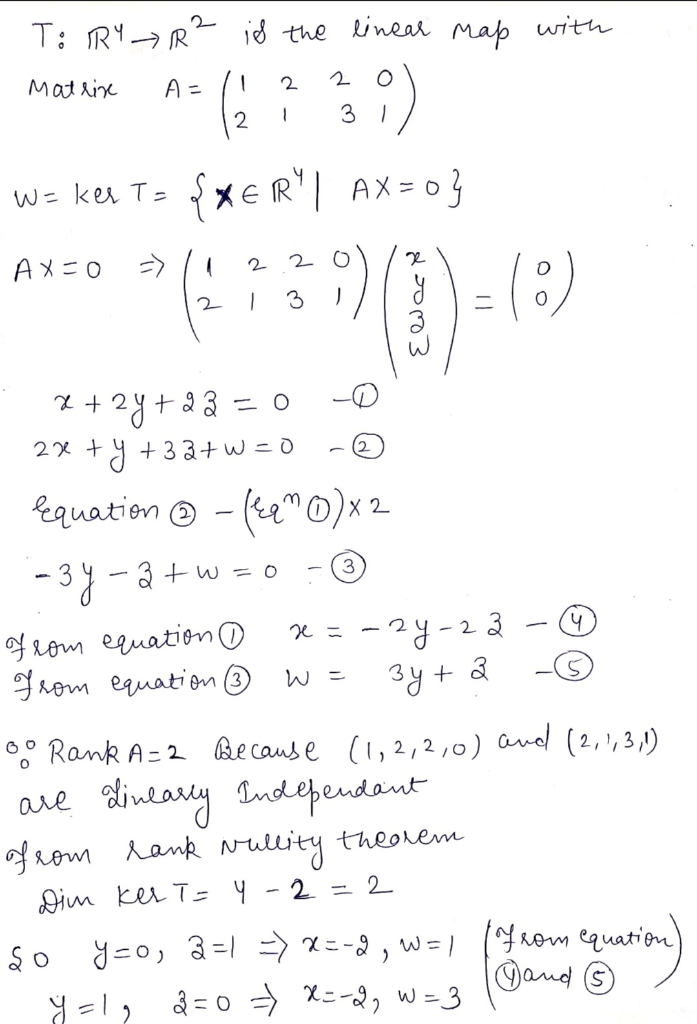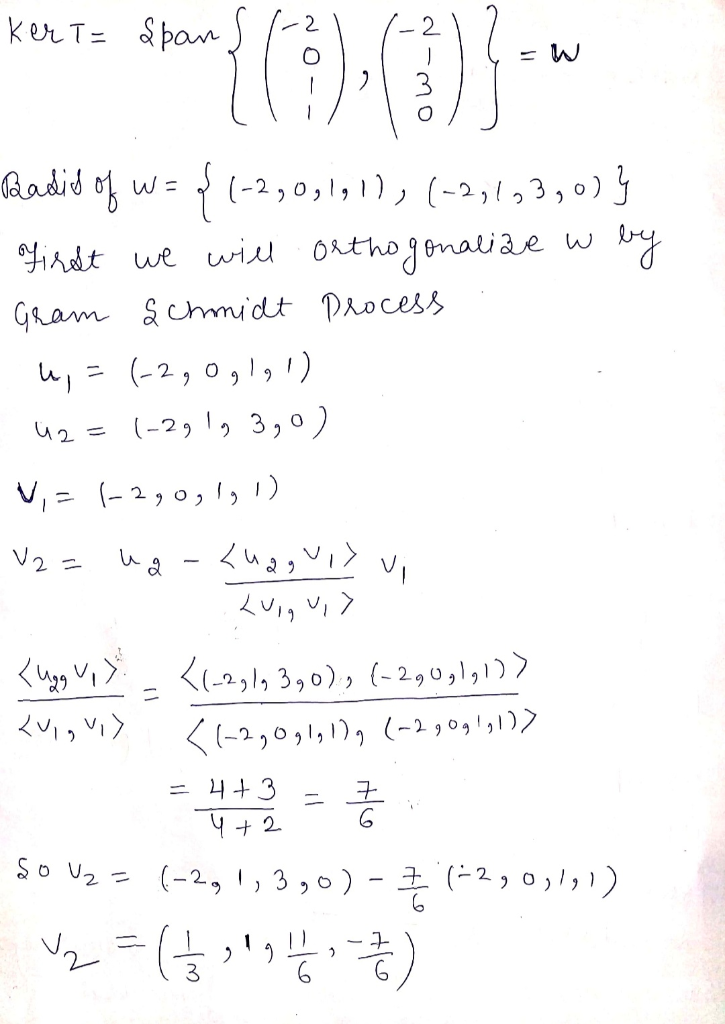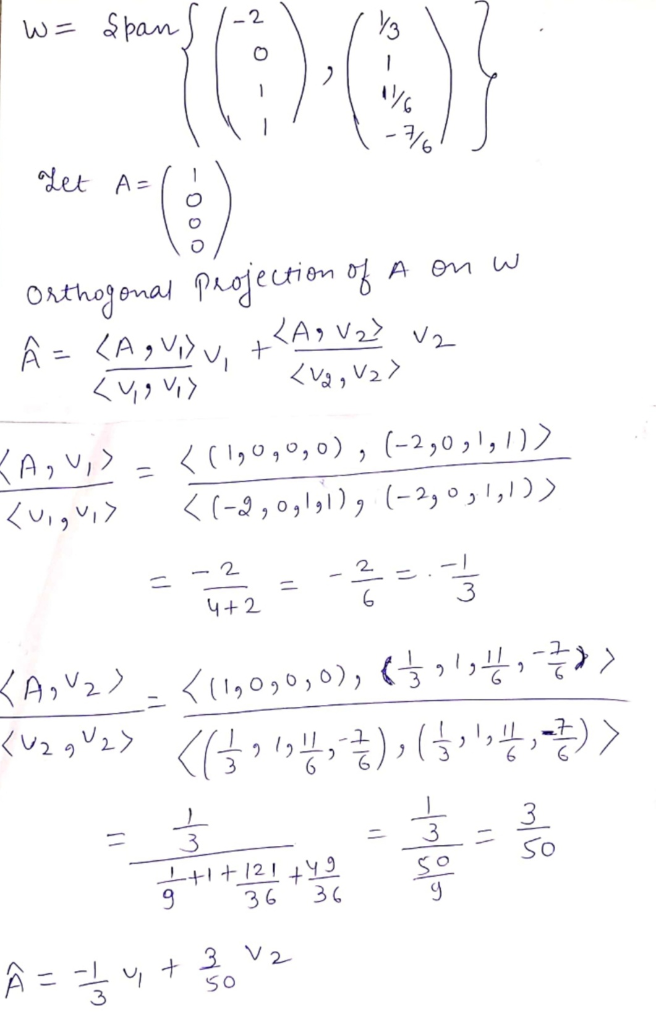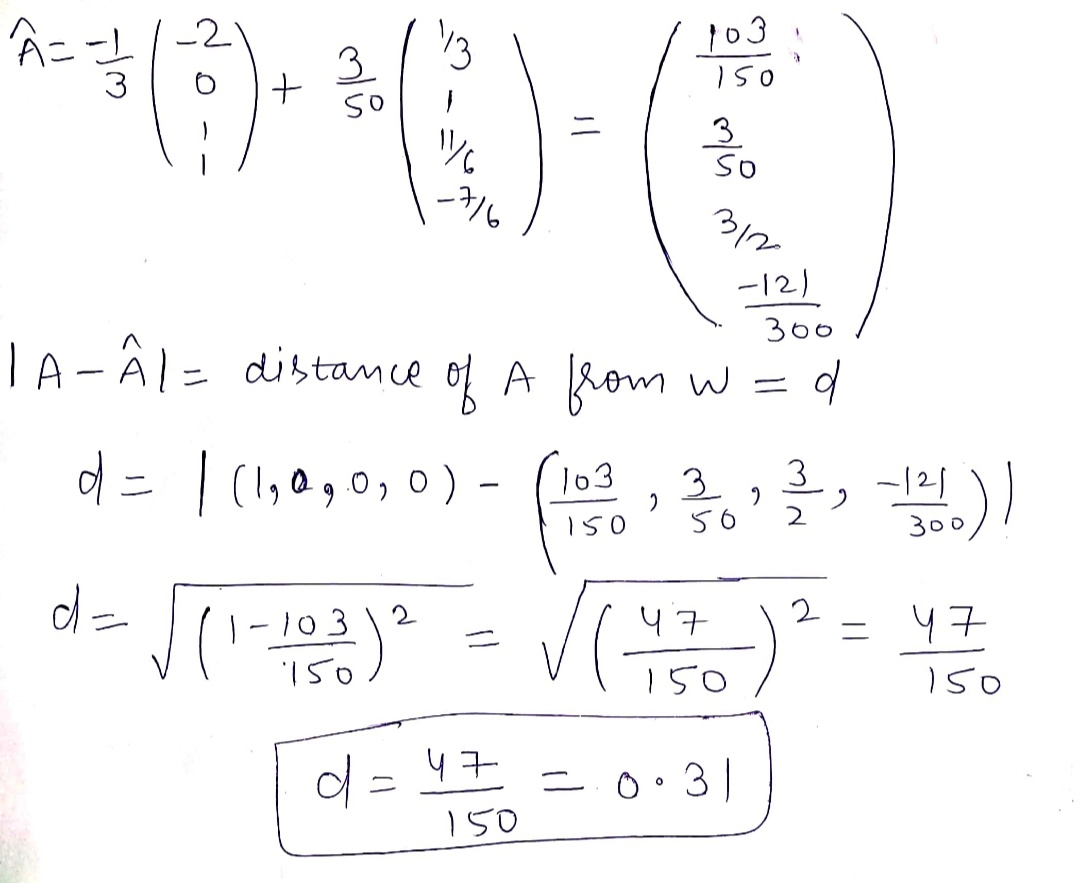# Work out the distance of the point [1, 0, 0, 0] from the subspace W =...

Work out the distance of the point [1, 0, 0, 0] from the subspace W = ker T, where T : R 4 → R 2 is the linear map with matrix A = {(1,2,2,0), (2,1,3,1)}

## Solutions

##### Expert Solution## Related Solutions

##### Compute the distance from the point x = (-5,5,2) to the subspace W of R3 consisting...
Compute the distance from the point x = (-5,5,2) to the subspace W of R3 consisting of all vectors orthogonal to the vector (1, 3, -2).
##### Let W be a subspace of Rn. Prove that W⊥ is also a subspace of Rn.
Let W be a subspace of Rn. Prove that W⊥ is also a subspace of Rn.
##### Use Lagrange multipliers to find the distance from the point (2, 0, −1) to the plane...
Use Lagrange multipliers to find the distance from the point (2, 0, −1) to the plane 4x − 3y + 8z + 1 = 0.
##### The equation 2x1-3x2=5 defines a line in R2. a. Find the distance from the point w=(3,1)...
The equation 2x1-3x2=5 defines a line in R2. a. Find the distance from the point w=(3,1) to the line by using projection. b. Find the point on the line closest to w by using the parametric equation of the line through w with vector a.
##### Determine whether or not W is a subspace of R3 where W consists of all vectors...
Determine whether or not W is a subspace of R3 where W consists of all vectors (a,b,c) in R3 such that 1. a =3b 2. a <= b <= c 3. ab=0 4. a+b+c=0 5. b=a2 6. a=2b=3c
##### Let W be a subspace of R^n, and P the orthogonal projection onto W. Then Ker...
Let W be a subspace of R^n, and P the orthogonal projection onto W. Then Ker P is W^perp.
##### Determine whether or not W is a subspace of V. Justify your answer. W = {p(x)...
Determine whether or not W is a subspace of V. Justify your answer. W = {p(x) ∈ P(R)|p(1) = −p(−1)}, V = P(R)
##### A particle moves from point A = (0, 0, 0) to point B = (2π, 0,...
A particle moves from point A = (0, 0, 0) to point B = (2π, 0, 2π), under the action of the force F = xi + yj − zk . a. Calculate the work done by the force F on the particle if it moves along the conic-helical curve r(t) = (t cost )i + (t sint )j + tk with 0 ≤ t ≤ 2π. b. Find a parametric vector equation for the straight line connecting A to...
##### # O W L S f(O,W,L,S) 0 0 0 0 0 0 1 0 0 0...
# O W L S f(O,W,L,S) 0 0 0 0 0 0 1 0 0 0 1 0 2 0 0 1 0 1 3 0 0 1 1 1 4 0 1 0 0 0 5 0 1 0 1 1 6 0 1 1 0 1 7 0 1 1 1 X 8 1 0 0 0 0 9 1 0 0 1 0 10 1 0 1 0 0 11 1 0 1 1 1 12 1...
##### If V (dimension k-1) is a subspace of W (dimension K), and V has an orthonormal...
If V (dimension k-1) is a subspace of W (dimension K), and V has an orthonormal basis {v1,v2.....vk-1}. Work out a orthonormal basis of W in terms of that of V and the orthogonal complement of V in W. Provide detailed reasoning.